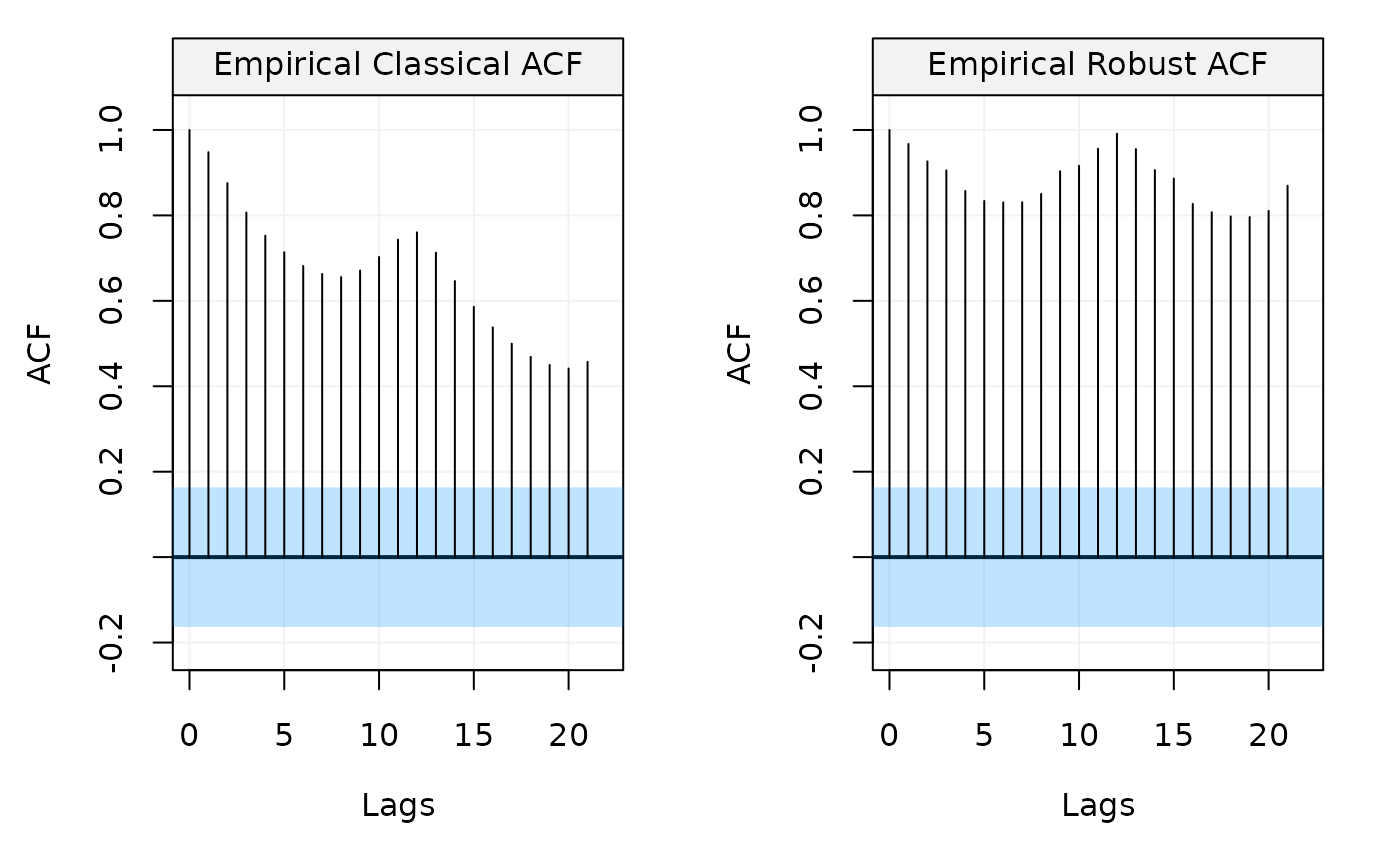Compare classical and robust ACF of univariate time series.

compare_acf(
x,
lag.max = NULL,
demean = TRUE,
show.ci = TRUE,
alpha = 0.05,
plot = TRUE,
...
)

## Arguments

x

A vector or "ts" object (of length $$N > 1$$).

lag.max

A integer indicating the maximum lag up to which to compute the ACF and PACF functions.

demean

A bool indicating whether the data should be detrended (TRUE) or not (FALSE). Defaults to TRUE.

show.ci

A bool indicating whether to compute and show the confidence region. Defaults to TRUE.

alpha

A double indicating the level of significance for the confidence interval. By default alpha = 0.05 which gives a 1 - alpha = 0.95 confidence interval.

plot

A bool indicating whether a plot of the computed quantities should be produced. Defaults to TRUE.

...

# Estimate both the ACF and PACF functions